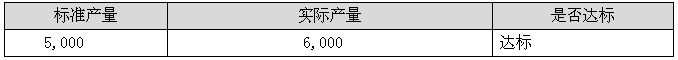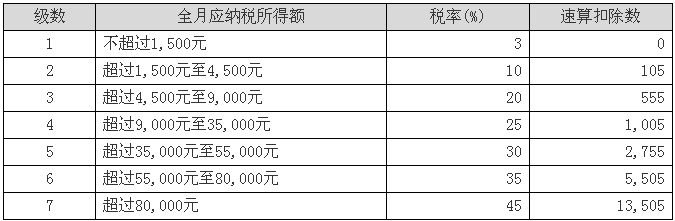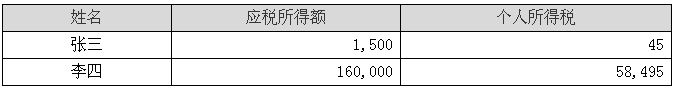EXCEL中使用频率最高的函数之一 IF函数使用介绍

IF函数是EXCEL中使用频率最高的函数之一，它通过一个条件判断返回TRUE（真）或FALSE（假）的结果。=IF(B2<5000,"不达标","达标")

=IF(B2<5000,"不达标",IF(B2<7000,"达标","超产")）C2单元格公式为：

=IF(B2<=1500,B2*3%-0,IF(B2<=4500,B2*10%-105,IF(B2<=9000,B2*20%-555,IF(B2<=35000,B2*25%-1005,IF(B2<=55000,B2*30%-2755,IF(B2<=80000,B2*35%-5505,B2*45%-13505))))))

tag : IF函数